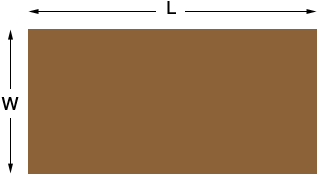SEARCH HOMEMath Central Quandaries & QueriesQuestion from Cynthia: a laundry room is shaped like a rectangle. the area of the room is 6 square yards. the perimeter is 10 yards. The room is longer than it is wide. How wide is the room? How long it the room?Hi Cynthia,I let the length be L yards and the width be W yards. Since the area is 6 square yards

L × W = 6.                            (1)

The perimeter is 10 yards so L + W + L + W = 10 or

L + W = 5.                            (2)

Solve equation (2) for L and substitute into equation (1). This will give you a quadratic equation in W which you can solve.

I hope this helps,
PennyMath Central is supported by the University of Regina and The Pacific Institute for the Mathematical Sciences.